Here we are providing Quadrilaterals Class 9 Extra Questions Maths Chapter 8 with Answers Solutions, Extra Questions for Class 9 Maths was designed by subject expert teachers. https://ncertmcq.com/extra-questions-for-class-9-maths/

Extra Questions for Class 9 Maths Chapter 8 Quadrilaterals with Solutions Answers

Quadrilateral Class 9 Extra Questions Question 1.
If one angle of a parallelogram is twice of its adjacent angle, find the angles of the parallelogram.
Solution:
Let the two adjacent angles be x and 2x.
In a parallelogram, sum of the adjacent angles are 180°
∴ x + 2x = 180°
⇒ 3x = 180°
⇒ x = 60°
Thus, the two adjacent angles are 120° and 60°. Hence, the angles of the parallelogram are 120°, 60°, 120° and 60°.

Class 9 Quadrilaterals Extra Questions Question 2.
If the diagonals of a quadrilateral bisect each other at right angles, then name the
Solution:
Rhombus.

Quadrilaterals Class 9 Extra Questions With Solutions Question 3.
Three angles of a quadrilateral are equal and the fourth angle is equal to 144o. Find each of the equal angles of the quadrilateral.
Solution:
Let each equal angle of given quadrilateral be x.
We know that, sum of all interior angles of a quadrilateral is 360°
∴ x + x + x + 144° = 360°
3x = 360° – 144°
3x = 216°
x = 72°
Hence, each equal angle of the quadrilateral is of 72o measures.

Extra Questions On Quadrilaterals Class 9 Question 4.
If ABCD is a parallelogram, then what is the measure of ∠A – ∠C ?
Solution:
∠A – ∠C = 0° (opposite angles of parallelogram are equal]

Quadrilaterals Class 9 Extra Questions Question 5.
PQRS is a parallelogram, in which PQ = 12 cm and its perimeter is 40 cm. Find the length of each side of the parallelogram.
Solution: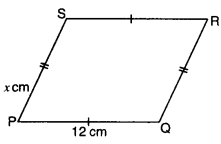Here, PQ = SR = 12 cm
Let PS = x and PS = QR
∴ x + 12 + x + 12 = Perimeter
2x + 24 = 40
2x = 16
x= 8
Hence, length of each side of the parallelogram is 12 cm, 8 cm, 12 cm and 8 cm.

Class 9 Maths Quadrilaterals Extra Questions Question 6.
Two consecutive angles of a parallelogram are (x + 60)° and (2x + 30)°. What special name can you give to this parallelogram?
Solution:
We know that consecutive interior angles of a parallelogram are supplementary.
∴ (x + 60° + (2x + 30)° = 180°
⇒ 3x° + 90° = 180°
⇒ 3x° = 90°
⇒ x° = 30°
Thus, two consecutive angles are (30 + 60)°, 12 x 30 + 30)”. i.e., 90° and 90°.
Hence, the special name of the given parallelogram is rectangle.

Class 9 Quadrilaterals Extra Questions Pdf Question 7.
ONKA is a square with ∠KON = 45°. Determine ∠KOA.
Solution:
Since ONKA is a square
∴ ∠AON = 90°
We know that diagonal of a square bisects its ∠s
⇒ ∠AOK = ∠KON = 45°
Hence, ∠KOA = 45°

Quadrilaterals Extra Questions Class 9 Question 8.
In quadrilateral PQRS, if ∠P = 60° and ∠Q : ∠R : ∠S = 2 : 3 : 7, then find the measure of ∠S.
Solution:
Let ∠Q = 2x, ∠R = 3x and ∠S = 7x
Now, ∠P + ∠Q + ∠R + ∠S = 360°
⇒ 60° + 2x + 3x + 7x = 360°
⇒ 12x = 300°
x = $$\frac{300^{\circ}}{12}$$ = 25°
∠S = 7x = 7 x 25° = 175°

Questions On Quadrilaterals For Class 9 Question 1.
ABCD is a parallelogram in which ∠ADC = 75° and side AB is produced to point E as shown in the figure. Find x + y.
Solution: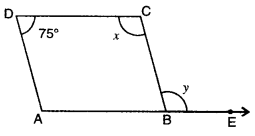Here, ∠C and ∠D are adjacent angles of the parallelogram.
∴ ∠C + ∠D = 180°
⇒ x + 75° = 180°
⇒ x = 105°
Also, y = x = 105° [alt. int. angles]
Thus, x + y = 105° + 105° = 210°

Class 9 Maths Chapter 8 Extra Questions With Solutions Question 2.
If the diagonals of a parallelogram are equal, then show that it is a rectangle.
Solution: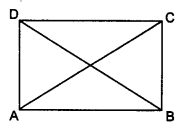Given: A parallelogram ABCD, in which AC = BD.
To Prove: ΔBCD is a rectangle.
Proof : In ΔABC and ΔBAD
AB = AB (common]
AC = BD (given]
BC = AD(opp. sides of a ||gm]
[by SSS congruence axiom]
Also, ∠ABC + ∠BAD = 180° (co-interior angles)
∠ABC + ∠ABC = 180° [ ∵ ∠ABC = ∠BAD ]
2∠ABC = 180°
∠ABC = 1/2 x 180° = 90°
Hence, parallelogram ABCD is a rectangle.

Quadrilateral Extra Questions Class 9 Question 3.
In the figure, ABCD is a rhombus, whose diagonals meet at O. Find the values of x and y.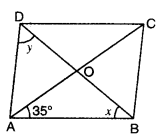Solution:
Since diagonals of a rhombus bisect each other at right angle.
In ∴ ΔAOB, we have
∠OAB + ∠x + 90° = 180°
∠x = 180° – 90° – 35°
= 55°
Also,
∠DAO = ∠BAO = 35°
∠y + ∠DAO + ∠BAO + ∠x = 180°
⇒ ∠y + 35° + 35° + 55° = 180°
⇒ ∠y = 180° – 125o = 55°
Hence, the values of x amd y are x = 55°, y = 55°.

Extra Questions For Class 9 Maths Quadrilaterals With Solutions Question 4.
ABCD is a parallelogram and AP and CQ are perpendiculars from vertices A and C on diagonal BD (see fig.). Show that :
(i) ΔAPB = ΔCQD
(ii) AP = CQ
Solution: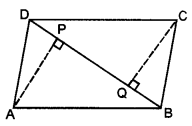Given : In ||gm ABCD, AP and CQ are perpendiculars from the
vertices A and C on the diagonal BD.
To Prove: (i) ΔAPB ≅ ΔCQD
(ii) AP = CQ
Proof : (i) In ΔAPB and ΔCQD
AB = DC (opp. sides of a ||gm ABCD]
∠APB = ∠DQC (each = 90°)
∠ABP = ∠CDQ (alt. int. ∠s]
⇒ ΔAPB ≅ ΔCQD[by AAS congruence axiom]
(ii) ⇒ AP = CQ [c.p.c.t.]

Extra Questions Of Quadrilaterals Class 9 Question 1.
The diagonals of a quadrilateral ABCD are perpendicular to each other. Show that the quadrilateral formed by joining the mid-points of its sides is a rectangle.Solution:
Given: A quadrilateral ABCD whose diagonals AC and BD are perpendicular to each other at O. P, Q, R and S are mid-points of side AB, BC, CD and DA respectively are joined are formed quadrilateral PQRS.
To Prove: PQRS is a rectangle.
Proof : In ∆ABC, P and Q are mid-points of AB and BC respectively.
∴ PQ || AC and PQ = $$\frac{1}{2}$$ AC … (i) (mid-point theorem]
Further, in SACD, R and S are mid-points of CD and DA respectively.
SR || AC and SR = $$\frac{1}{2}$$ AC … (ii) (mid-point theorem]
From (i) and (ii), we have PQ || SR and PQ = SR
Thus, one pair of opposite sides of quadrilateral PQRS are parallel and equal.
∴ PQRS is a parallelogram.
Since PQ|| AC PM || NO
In ∆ABD, P and S are mid-points of AB and AD respectively.
PS || BD (mid-point theorem]
⇒ PN || MO
∴ Opposite sides of quadrilateral PMON are parallel.
∴ PMON is a parallelogram.
∠ MPN = ∠ MON (opposite angles of ||gm are equal]
But ∠MON = 90° [given]
∴ ∠MPN = 90° ⇒ ∠QPS = 90°
Thus, PQRS is a parallelogram whose one angle is 90°
∴ PQRS is a rectangle.

Class 9 Maths Chapter 8 Extra Questions Question 2.
In the fig., D, E and F are, respectively the mid-points of sides BC, CA and AB of an equilateral triangle ABC. Prove that DEF is also an equilateral triangle.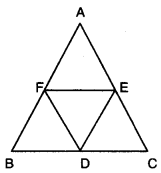Solution:
Since line segment joining the mid-points of two sides of a triangle is half of the third side.
Therefore, D and E are mid-points of BC and AC respectively.
⇒ DE = $$\frac{1}{2}$$AB …(i)
E and F are the mid-points of AC and AB respectively.
∴ EF = $$\frac{1}{2}$$BC … (ii)
F and D are the mid-points of AB and BC respectively.
∴ FD = $$\frac{1}{2}$$ AC … (iii)
Now, SABC is an equilateral triangle.
⇒ AB = BC = CA
⇒ $$\frac{1}{2}$$AB = $$\frac{1}{2}$$BC = $$\frac{1}{2}$$CA
⇒ DE = EF = FD (using (i), (ii) and (iii)]
Hence, DEF is an equilateral triangle.

Quadrilateral Class 9 Questions Question 3.
In quadrilateral ABCD of the given figure, X and Y are points on diagonal AC such that AX = CY and BXDY is a parallelogram. Show that ABCD is a parallelogram.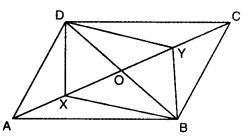Solution:
Since BXDY is a parallelogram.
XO = YO
DO = BO
[∵ diagonals of a parallelogram bisect each other]
But AX = CY …. (iii) (given]
Adding (i) and (iii), we have
XO + AX = YO + CY
⇒ AO = CO …. (iv)
From (ii) and (iv), we have
AO = CO and DO = BO
Thus, ABCD is a parallelogram, because diagonals AC and BD bisect each other at O.

Class 9 Maths Ch 8 Extra Questions Question 4.
ABCD is a quadrilateral in which the bisectors of ∠A and ∠C meet DC produced at Y and BA produced at X respectively. Prove that
∠X +∠Y = $$\frac{1}{2}$$(∠A + ∠C)
Solution: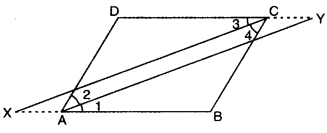Here, ∠1 = ∠2 and ∠3 = ∠4
In ΔXBC, we have
∠X + ∠B + ∠4 = 180°
∠X + ∠B + $$\frac{1}{2}$$∠C = 180
∠2 + ∠D + ∠Y= 180°
$$\frac{1}{2}$$ ∠A + ∠D + ∠Y = 180°
Adding (i) and (ii), we have
∠X + ∠Y + ∠B + ∠D + $$\frac{1}{2}$$ ∠C + $$\frac{1}{2}$$ ∠A = 360°
∠A + ∠B + ∠C + ∠D = 360°
∠X + ∠Y + ∠B + ∠D + $$\frac{1}{2}$$ ∠C + $$\frac{1}{2}$$ ∠A = ∠A + ∠B + ∠C + ∠D
∠X + ∠Y = ∠A – $$\frac{1}{2}$$ ∠A + $$\frac{1}{2}$$ ∠C – $$\frac{1}{2}$$ ∠C
∠X+ ∠Y = $$\frac{1}{2}$$ (∠A + ∠C)

Quadrilateral Questions For Class 9 Question 1.
In the figure, P, Q and R are the mid-points of the sides BC, AC and AB of ΔABC. If BQ and PR intersect at X and CR and PQ intersect at Y, then show that XY = $$\frac{1}{4}$$ BC.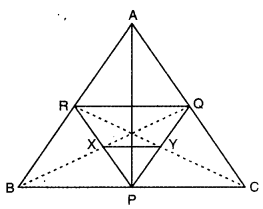Solution:
Here, in ΔABC, R and Q are the mid-points of AB and AC respectively.
∴ By using mid-point theorem, we have
RQ || BC and RQ = $$\frac{1}{2}$$ BC
∴ RQ = BP = PC [∵ P is the mid-point of BC]
∴ RQ || BP and RQ || PC
RQ || BP, RQ = BP (proved above]
∴ BPQR is a parallelogram. [∵ one pair of opp. sides is parallel as well as equal]
∴ X is the mid-point of PR. [∵ diagonals of a ||gm bisect each other]
RQ || PC and RQ = PC [proved above)
∴ PCQR is a parallelogram [∵ one pair of opp. sides is parallel as well as equal]
∴ Y is the mid-point of PQ [∵ diagonals of a ||gm bisect each other]
In ΔPQR
∴ X and Y are mid-points of PR and PQ respectively.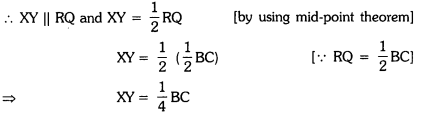Class 9 Quadrilaterals Important Questions Question 2.
In the given figure, AE = DE and BC || AD. Prove that the points A, B, C and D are concyclic. Also, prove that the diagonals of the quadrilateral ABCD are equal.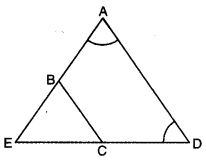Solution:
Since AE = DE
∠D = ∠A …. (i) [∵ ∠s opp. to equal sides of a Δ]
∠EBC = ∠A …. (ii) (corresponding ∠s]
From (i) and (ii), we have
∠D = ∠EBC …. (iii)
But ∠EBC + ∠ABC = 180° (a linear pair]
∠D + ∠ABC = 180° (using (iii)]
Now, a pair of opposite angles of quadrilateral ABCD is supplementary
Thus, ABCD is a cyclic quadrilateral i.e., A, B, C and D’are concyclic. In ΔABD and ΔDCA
∠ABD = ∠ACD [∠s in the same segment for cyclic quad. ABCD]
So, by using AAS congruence axiom, we have
ΔABD ≅ ΔDCA
Hence, BD = CA [c.p.c.t.]

Question 3.
In ΔABC, AB = 8 cm, BC = 9 cm and AC = 10 cm. X, Y and Z are mid-points of AO, BO and CO respectively as shown in the figure. Find the lengths of the sides of ΔXYZ.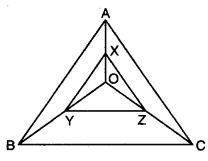Solution:
Here, in ΔABC, AB = 8 cm, BC = 9 cm, AC = 10 cm.
In ΔAOB, X and Y are the mid-points of AO and BO.
∴ By using mid-point theorem, we have
XY = $$\frac{1}{2}$$ AB = $$\frac{1}{2}$$ x 8 cm = 4 cm
Similarly, in Δ𝜏BOC, Y and Z are the mid-points of BO and CO.
∴ By using mid-point theorem, we have
YZ = $$\frac{1}{2}$$ BC = $$\frac{1}{2}$$ x 9cm = 4.5 cm
And, in Δ𝜏COA, Z and X are the mid-points of CO and AO.
∴ ZX = $$\frac{1}{2}$$ AC = $$\frac{1}{2}$$ x 10 cm = 5 cm
Hence, the lengths of the sides of ΔXYZ are XY = 4 cm, YZ = 4.5 cm and ZX = 5 cm.

Question 4.
PQRS is a square and ∠ABC = 90° as shown in the figure. If AP = BQ = CR, then prove that ∠BAC = 45°Solution:
Since PQRS is a square.
∴ PQ = QR … (I) [∵ sides of a square are equal]
Also, BQ = CR … (ii) [given]
Subtracting (ii) from (i), we obtain
PQ – BQ = QR – CR
⇒ PB = QC … (iii)
In Δ𝜏APB and Δ𝜏BQC
AP = BQ[given
∠APB = ∠BQC = 90°](each angle of a square is 90°)
PB = QC (using (iii)]

So, by using SAS congruence axiom, we have
ΔAPB ≅ ΔBQC
∴ AB = BC [c.p.c.t.]
Now, in ΔABC
AB = BC [proved above]
∴ ∠ACB = ∠BAC = x° (say) [∠s opp. to equal sides]
Also, ∠B + ∠ACB + ∠BAC = 180°
⇒ 90° + x + x = 180°
⇒ 2x° = 90°
x° = 45°
Hence, ∠BAC = 45°

Question 5.
ABCD is a parallelogram. If the bisectors DP and CP of angles D and C meet at P on side AB, then show that P is the mid-point of side AB.
Solution:Since DP and CP are angle bisectors of ∠D and ∠C respectively.
: ∠1 = ∠2 and ∠3 = ∠4
Now, AB || DC and CP is a transversal
∴ ∠5 = ∠1 [alt. int. ∠s]
But ∠1 = ∠2 [given]
∴ ∠5 = ∠2

Now, in ABCP, ∠5 = ∠2
⇒ BC = BP … (I) [sides opp. to equal ∠s of a A]
Again, AB || DC and DP is a transversal.
∴ ∠6= ∠3 (alt. int. Δs]
But ∠4 = ∠3 [given]
∴ ∠6 = ∠4
Now, in ΔADP, ∠6 = ∠4
⇒ DA = AP …. (ii) (sides opp. to equal ∠s of a A]
Also, BC = DA… (iii) (opp. sides of parallelogram)
From (i), (ii) and (iii), we have
BP = AP
Hence, P is the mid-point of side AB.

Question 6.
In the figure, ΔBCD is a trapezium in which AB || DC. E and F are the mid-points of AD and BC respectively. DF and AB are produced to meet at G. Also, AC and EF intersect at the point O. Show that :
(i) EO || AB
(ii) AO = COSolution:
Here, E and F are the mid-points of AD and BC respectively.
In ΔBFG and ΔCFD
BF = CF [given]
∠BFG = ∠CFD (vert. opp. ∠s]
∠BGF = ∠CDF (alt. int. ∠s, as AB || DC)
So, by using AAS congruence axiom, we have
ΔBFG ≅ ΔCFD
⇒ DF = FG [c.p.c.t.)
Now, in ΔAGD, E and F are the mid-points of AD and GD.
∴ By mid-point theorem, we have
EF || AG
or EO || AB
Also, in ΔADC, EO || DC
∴ EO is a line segment from mid-point of one side parallel to another side.
Thus, it bisects the third side.
Hence, AO = CO